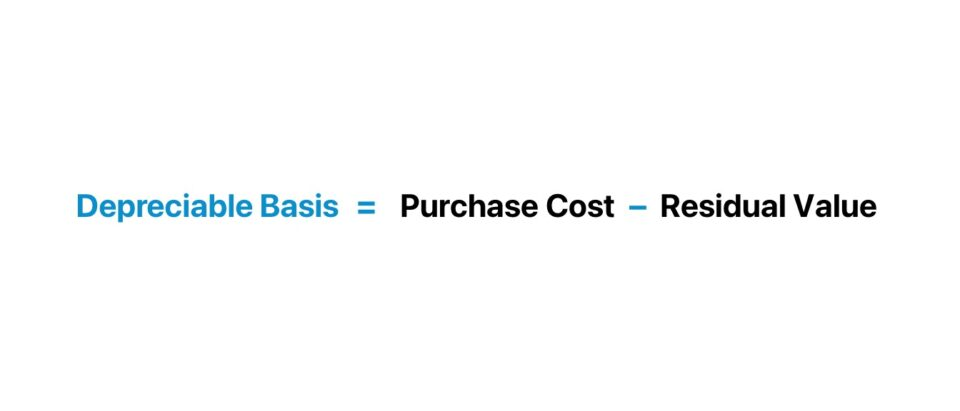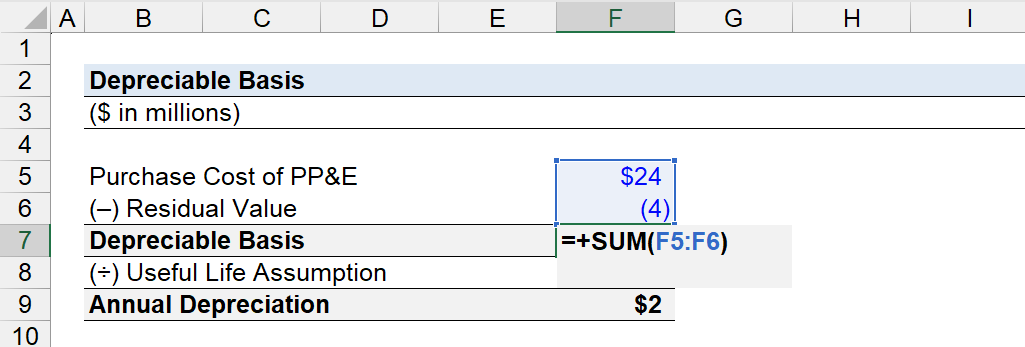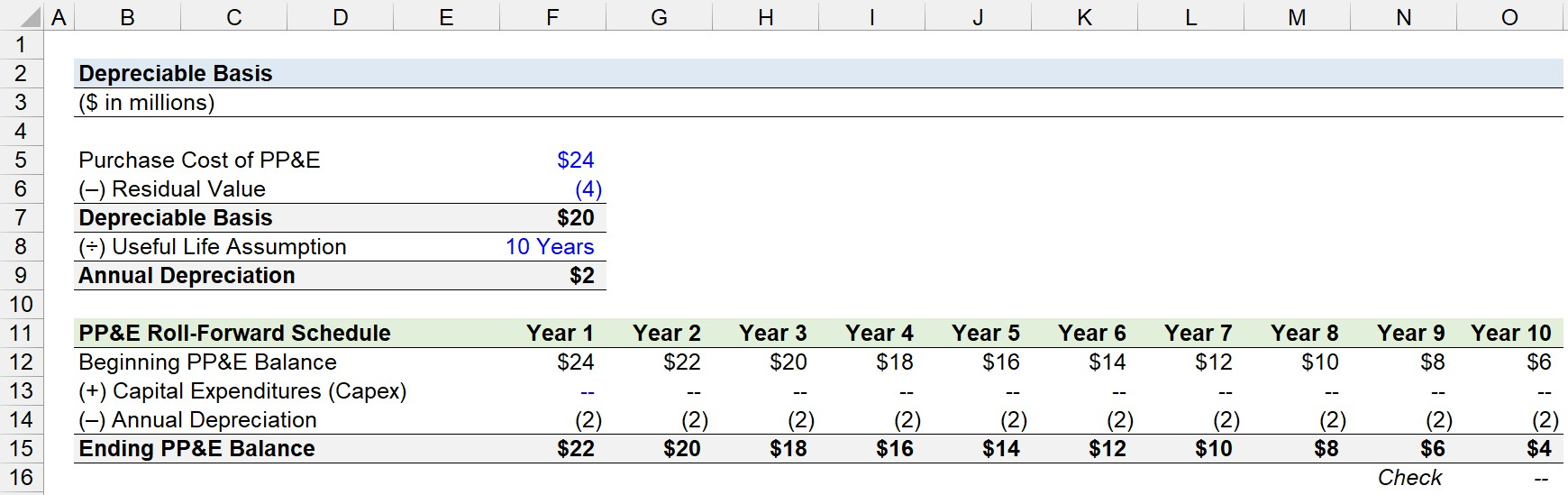Welcome to Wall Street Prep! Use code at checkout for 15% off.# Depreciable Basis

Guide to Understanding the Depreciable Base## How to Calculate Depreciable Basis?

The depreciable basis for a fixed asset (PP&E) is the total amount of depreciation that can be recognized on the income statement across the useful life of the fixed asset.

The depreciable basis, or “depreciation base”, equals the initial purchase cost of the fixed asset minus the salvage value (or “residual value”) assumption.

• Purchase Cost → The total cost incurred to acquire the fixed asset – i.e. the capital expenditure (Capex) – on the date of initial purchase. The total purchase cost must be adjusted to include any expenses incurred in addition, such as installation fees, testing costs, custom modifications, and other costs incurred to ensure the fixed asset provides the most amount of value based on the specific needs of the company.
• Salvage Value → The estimated residual value of the fixed asset remaining at the end of its useful life assumption, or the “scrap value” at which the fixed asset could be sold in the open markets.

In practice, the salvage value of the fixed asset is usually assumed to be zero at the end of its useful life.

Why? The higher the depreciation expense recorded on the income statement, the greater the tax savings for bookkeeping purposes (vs. for tax purposes).

In other words, a higher depreciation base results in a greater annual depreciation expense, which reduces the taxable income, or “pre-tax income (EBT)”, on the income statement.

By recognizing a higher amount of annual depreciation expense, the positive effects of the depreciation “tax shield” increase, whereby the taxable income is reduced (and causes the income taxes incurred to decrease).

The greater the depreciable base and the coinciding increase in the annual depreciation expense therefore cause net income and earnings per share (EPS) to rise – all else being equal.

## Depreciable Basis Formula

The formula used to calculate the depreciable basis is the difference between the purchase cost of a fixed asset (PP&E) minus its salvage value assumption.

Depreciable Basis = Purchase Cost of Fixed Asset  Salvage Value

Once the depreciable base is determined, the next step is to divide the depreciation base by the useful life assumption – i.e. the estimated number of years in which the fixed asset is expected to continue to provide positive economic utility on behalf of the company.

The annual depreciation expense is thus the depreciation base divided by the estimated useful life of the fixed asset.

Annual Depreciation Expense = Depreciable Basis ÷ Estimated Useful Life Assumption

The calculation of the annual depreciation expense, however, can become more intricate under different depreciation methods.

• Straight-Line Depreciation Method → The straight-line depreciation method recognizes depreciation in a uniform pattern, wherein the expense is “spread” evenly across the fixed asset’s useful life assumption. Compared to the other depreciation methods, the straight-line method tends to be more common among publicly traded companies, namely because of the convenience factor – which is the implicit assumption in our formula for annual depreciation.
• Accelerated Depreciation Method → Accelerated depreciation methods, such as the double declining method (DDM), allocate a greater percentage of the depreciation expense to the earlier stages of the fixed asset’s useful life based on the notion that the economic utility of the fixed asset wanes with time.
• Units of Production Method → The units of production method ties the depreciation expense to the usage (or production capacity) of the fixed asset.

## Depreciable Basis Calculator

We’ll now move on to a modeling exercise, which you can access by filling out the form below.#### Get the Excel Template!Submitting...

## 1. Depreciable Basis Calculation Example

Suppose a manufacturer acquired a fixed asset (PP&E) for \$24 million at the end of Year 0.

The residual value of the fixed asset is assumed to be \$4 million, while its useful life assumption is expected to be 10 years.

• Purchase Cost of PP&E = \$24 million
• Residual Value = \$4 million
• Useful Life = 10 Years

Given the purchase price of the fixed asset (PP&E) and the residual value assumption, we can determine the depreciable basis by deducting the residual value from the purchase cost of the fixed asset.

• Depreciable Basis = \$24 million – \$4 million = \$20 million## 2. PP&E Roll-Forward Schedule Build

In the next section, we’ll create a simple PP&E roll-forward schedule, where the beginning PP&E balance is adjusted by capital expenditures (Capex) and the annual depreciation.

Ending PP&E Balance = Beginning PP&E Balance + Capital Expenditures (Capex) Annual Depreciation

Starting off, we’ll link the purchase cost of the fixed asset (PP&E) into the “Beginning PP&E Balance” for Year 1, i.e. the \$24 million is the ending PP&E balance for Year 0.

In the “Capital Expenditures (Capex)” row, we’ll assume no additional Capex was incurred over the time-year time frame to better illustrate the concept.

Therefore, the depreciable basis of the fixed asset (PP&E) is \$20 million, which we’ll divide by the useful life assumption of 10 years to determine the annual depreciation expense.

• Annual Depreciation = \$20 million ÷ \$10 million = \$2 million

Since our model assumes the depreciation method is on a straight-line basis, the annual depreciation expense is linked to (and anchored via pressing F4) our PP&E roll-forward – i.e. via a constant reduction.

Therefore, the “Beginning PP&E Balance” is reduced by the “Annual Depreciation” each period until reaching Year 10, the end of the fixed asset’s useful life.

The “Ending PP&E Balance” is the “Beginning PP&E Balance” for each subsequent period, as part of standard roll-forward modeling mechanics.

Once complete, the “Ending PP&E Balance” at the end of Year 10 is \$4 million, which matches our residual value assumption of \$4 million.Step-by-Step Online Course

### Everything You Need To Master Financial Modeling

Enroll in The Premium Package: Learn Financial Statement Modeling, DCF, M&A, LBO and Comps. The same training program used at top investment banks.

Inline Feedbacks